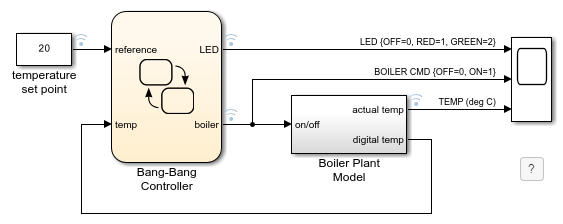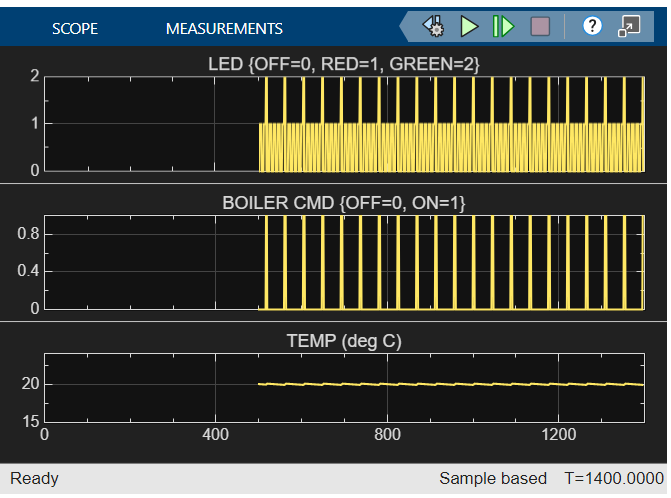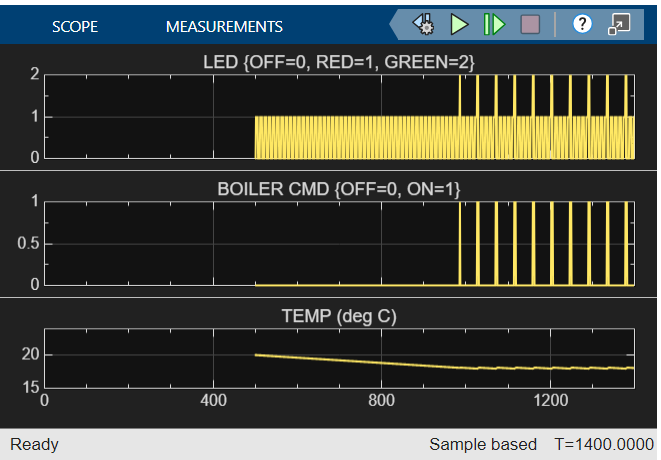## Use Operating Points to Specify Initial State of Simulation

This example shows how to use operating points to get the results of a continuous simulation without starting from time t = 0. An operating point is a snapshot of the state of a Simulink® model during simulation. If your model contains a Stateflow® chart, the operating point includes information about active states, output and local data, and persistent variables. For more information, see Save and Restore Operating Points for Stateflow Charts.

The `sf_boiler` model illustrates the logic of a bang-bang control system that regulates the temperature of a boiler.This model simulates for 1400 seconds. During the initial phase of the simulation, the temperature of the boiler rises from 15℃ to 20℃. This initial phase of the simulation takes approximately 500 seconds.To see how the system reacts to changes in the temperature set point after the initial phase of simulation, simulate the model and save the operating point at t =500. Then, load that operating point and run the simulation between t = 500 and t = 1400 using different temperature set points.

### Save Operating Point for Initial Simulation Segment

1. Open the `sf_boiler` model.

`openExample("stateflow/BangBangControlUsingTemporalLogicExample")`
2. Set the model to save the final operating point. Open the Configuration Parameters dialog box and, in the Data Import/Export pane:

1. Select Final states and enter a name for the operating point. For this example, use `xFinal`.

2. Select Save final operating point.

3. Click OK.

3. Set the stop time for this simulation segment. In the Simulation tab, set Stop Time to `500`.

4. Run the simulation.

When you simulate the model, you save the operating point at t = 500 in the variable `xFinal` in the MATLAB® base workspace.5. In the Configuration Parameters dialog box, in the Data Import/Export pane, clear the Save final operating point and Final states parameters. This step prevents you from overwriting the operating point you saved in the previous step.

### Start Simulation from Operation Point

1. Load the operating point as the initial state of the model. In the Configuration Parameters dialog box, in the Data Import/Export pane, select Initial state and enter the variable that contains the operating point of your chart, `xFinal`. Then, click OK.

2. Define the stop time for the new simulation segment. In the Simulation tab, set Stop Time to `1400`.

3. Run the simulation.

The model starts at t = 500 without repeating the initial phase of the simulation. The Scope block shows the chart output between t = 500 and t = 1400 using the original temperature set point of 20℃.4. Change the value of the `temperature set point` block to `18`.

5. Run the simulation again.

The Scope block shows the chart output between t = 500 and t = 1400 using the modified temperature set point of 18℃.